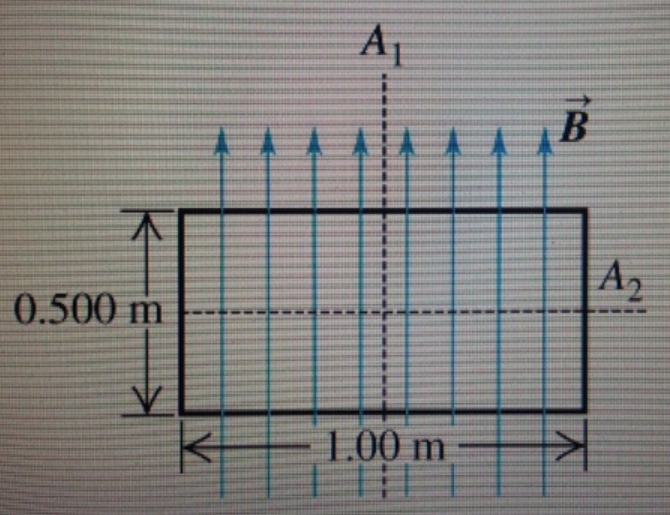# Problem: A uniform rectangular coil of total mass 270 g and dimensions 0.500m×1.00m is oriented parallel to a uniform 3.70-T magnetic field (Figure 1) . A current of 2.00 A is suddenly started in the coil.Find the initial angular acceleration of the coil just after the current is started.Express your answer using two significant figures.ANSWER = _________________ rad /S2

###### FREE Expert Solution

Magnetic torque:

$\overline{){\mathbf{\tau }}{\mathbf{=}}{\mathbf{N}}{\mathbf{B}}{\mathbf{A}}{\mathbf{i}}{\mathbf{·}}{\mathbf{s}}{\mathbf{i}}{\mathbf{n}}{\mathbf{\theta }}}$

Torque:

$\overline{){\mathbit{\tau }}{\mathbf{=}}{\mathbit{\alpha }}{\mathbit{I}}}$ where I is the moment of inertia and α is the angular acceleration.

Moment of inertia of a rod about its center:

$\overline{){\mathbf{I}}{\mathbf{=}}\frac{\mathbf{1}}{\mathbf{12}}{\mathbf{M}}{{\mathbf{L}}}^{{\mathbf{2}}}}$

Parallel axis theorem:

$\overline{){\mathbf{I}}{\mathbf{=}}{{\mathbf{I}}}_{\mathbf{c}\mathbf{m}}{\mathbf{+}}{\mathbf{M}}{{\mathbf{d}}}^{{\mathbf{2}}}}$

92% (102 ratings)###### Problem Details

A uniform rectangular coil of total mass 270 g and dimensions 0.500m×1.00m is oriented parallel to a uniform 3.70-T magnetic field (Figure 1) . A current of 2.00 A is suddenly started in the coil.

Find the initial angular acceleration of the coil just after the current is started.